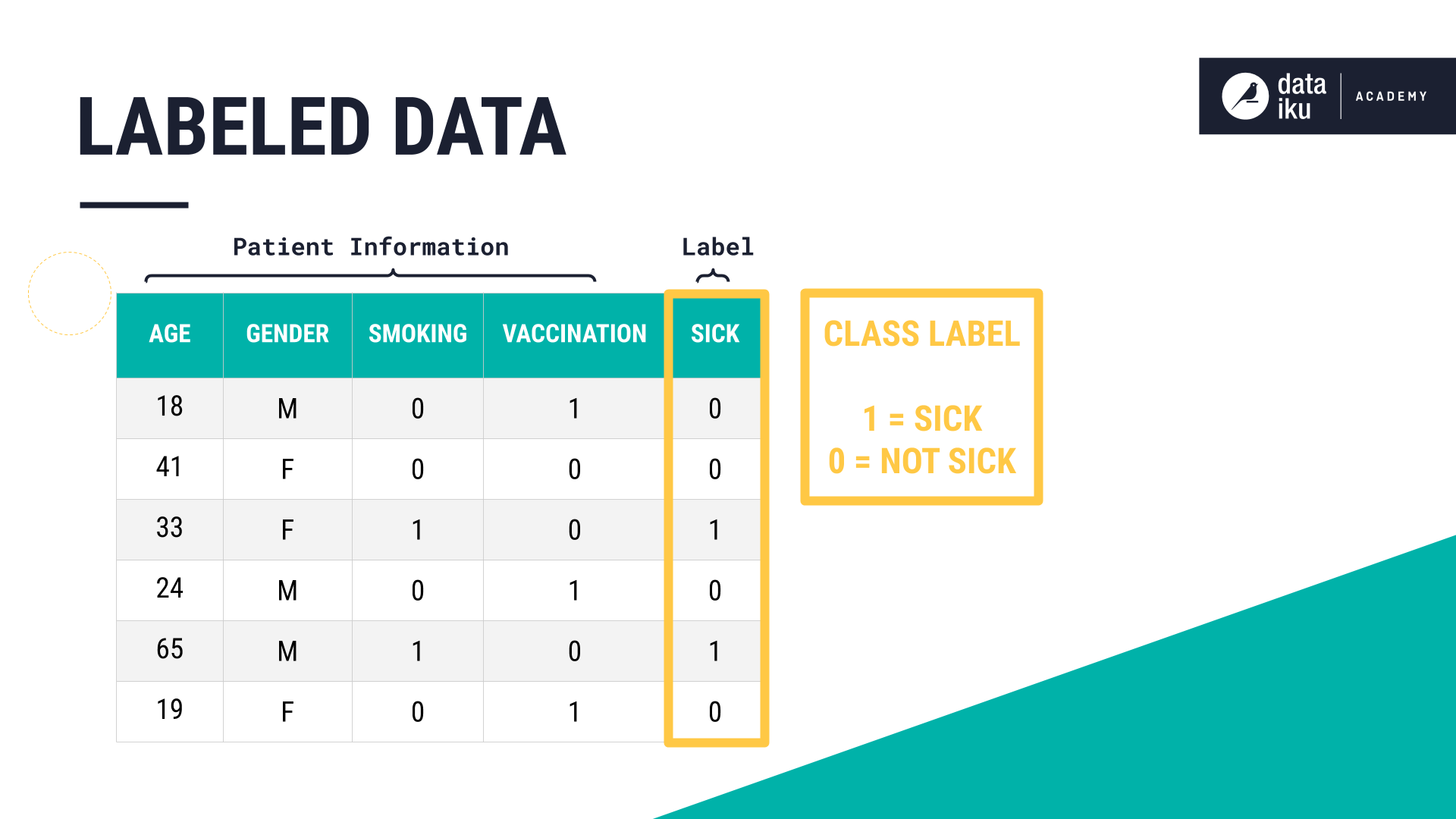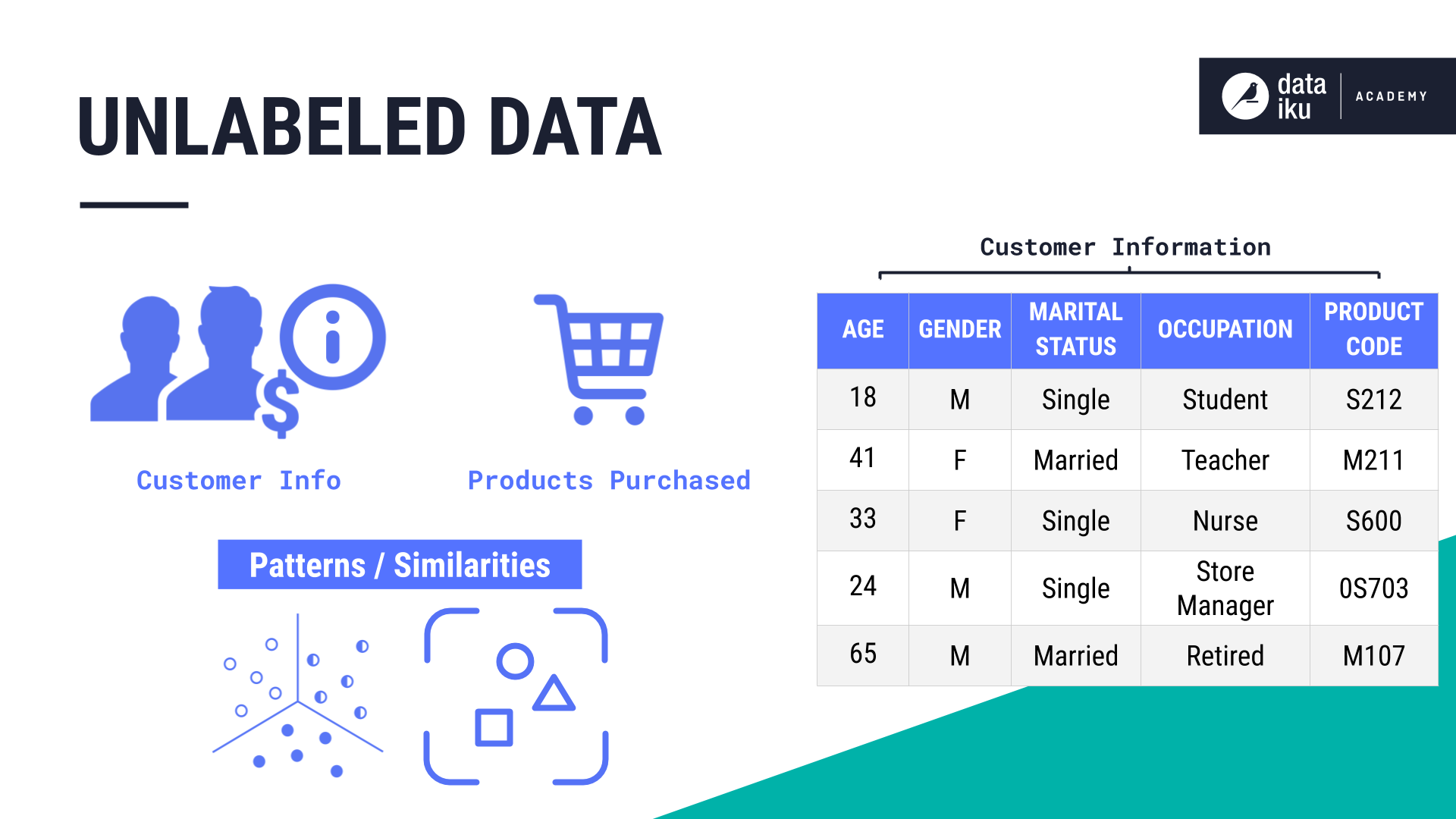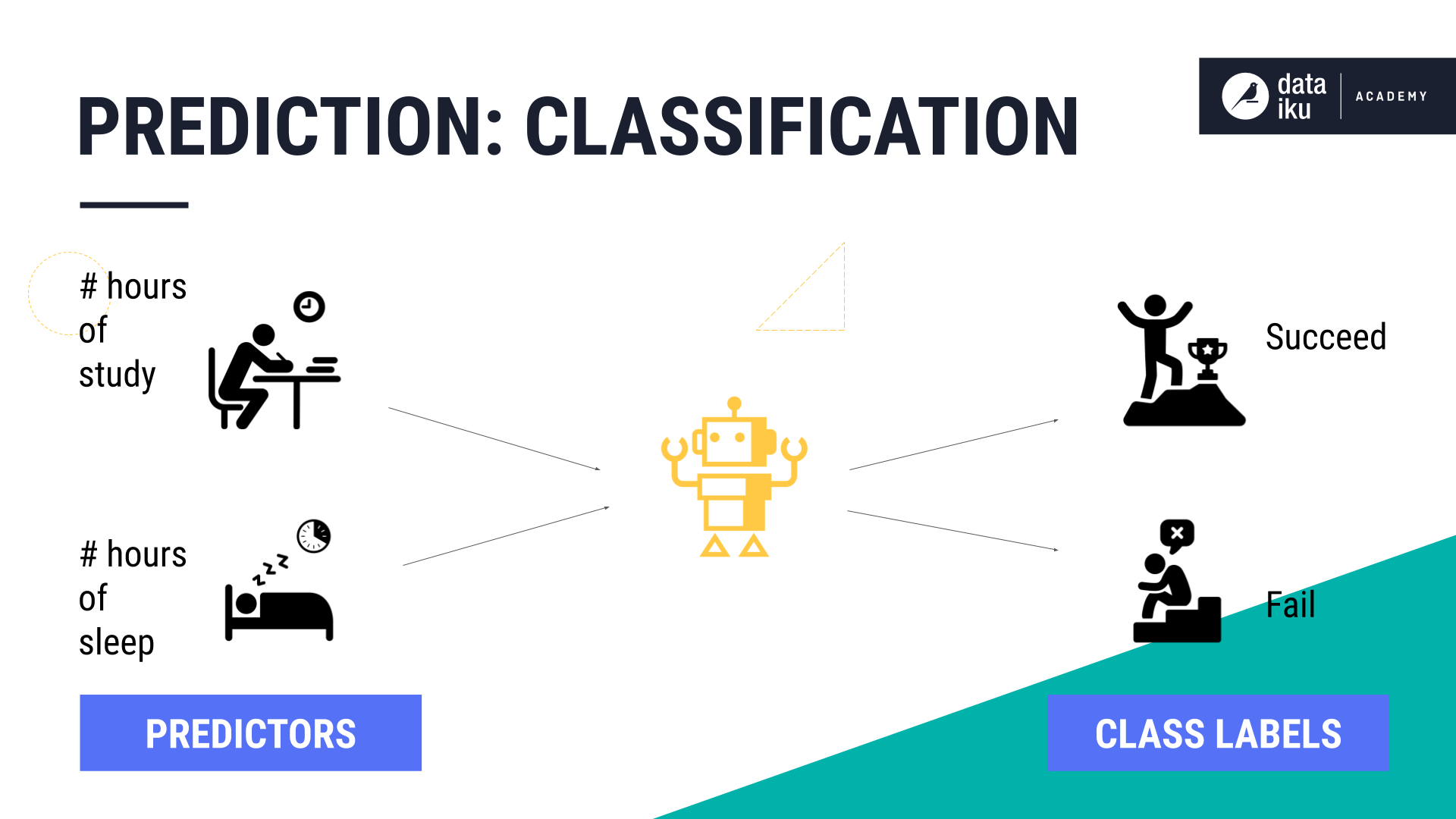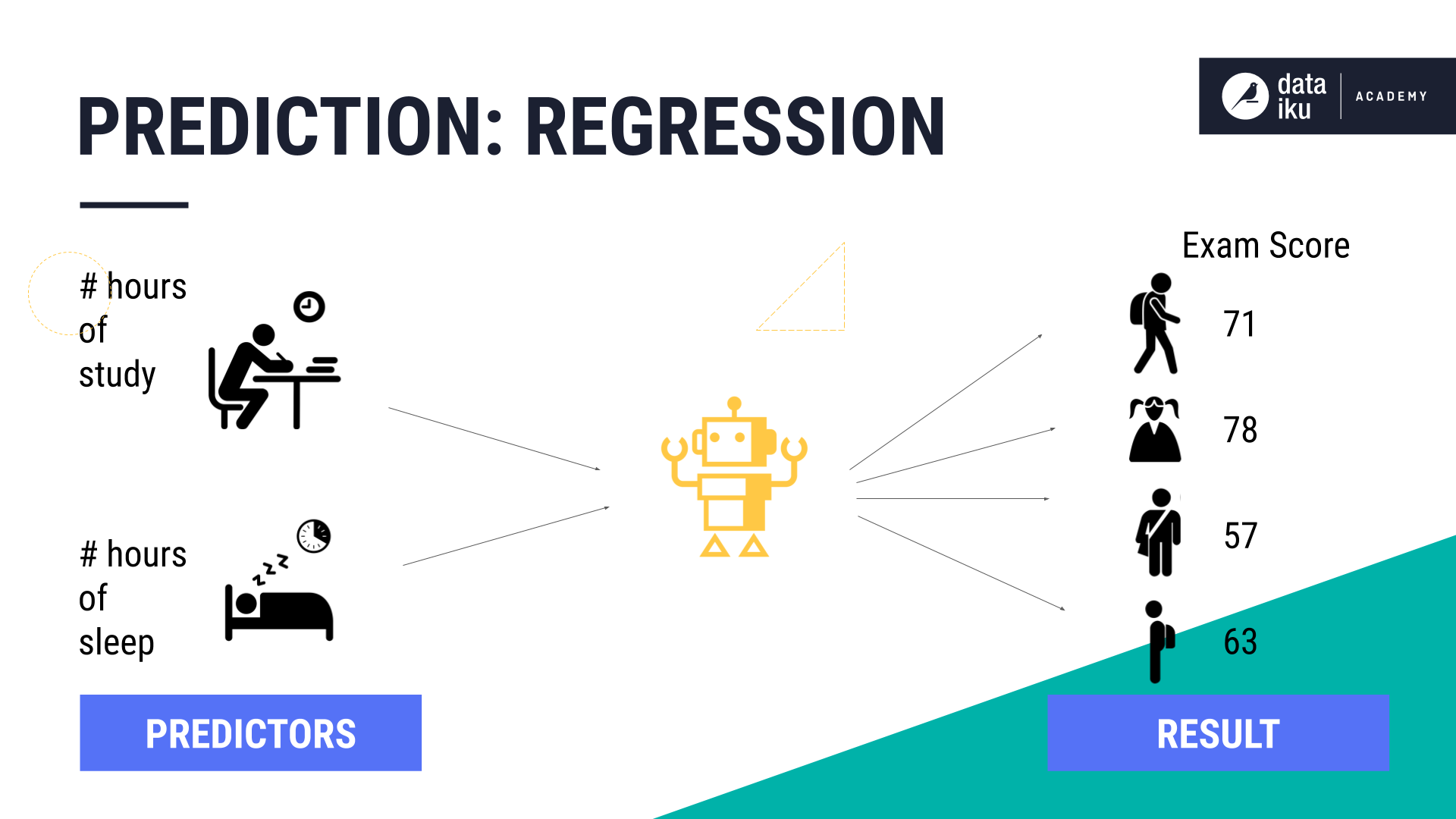# Concept Summary: Predictive Modeling¶

## What is Predictive Modeling?¶

With the availability of quick modeling tools, it is easy to jump in and start building machine learning models. This lesson introduces some basic concepts such as the difference between supervised and unsupervised learning, and regression and classification. Knowledge of these concepts may better prepare you for the challenges you are likely to face when you start to analyze your available data sources in preparation for building machine learning models.

To understand the difference between supervised and unsupervised learning, let’s look at labeled and unlabeled data.

This labeled data set contains patient information. The values in the class label column, Sick, represent an outcome, “sick or not sick”, where 1 means the patient is “sick.” Using this column, along with patient characteristics, also known as variables or features, such as age, gender, and smoking, we can teach the machine the relationship between the features and the resulting outcome. This approach falls under the category of prediction, also referred to as supervised learning.An unlabeled data set does not have a class label column that represents an outcome. In our example, we have an unlabeled data set containing information about customers, including which products they have purchased. Even though there is no class label, a machine can still learn about the customers by finding patterns and similarities in the data set using a learning method known as clustering, and it falls under the category of unsupervised learning.### Classification vs. Regression¶

Prediction problems can be either classification or regression.

In a classification problem, prediction means that we use some training data to understand how input variables (such as attributes of a patient) relate to the output. This output could be a class label, such as Sick or Not Sick. Having only two class labels is known as binary classification. The model is trained to understand the relationship between the input variables and the output variable (or class label).

In a regression problem, we predict a distinct value or a continuous value, given the input variables.

Let’s look at a use case to help illustrate the distinction. Consider that we want to understand how the number of sleep hours and study hours (the predictors) determine if a student will Succeed or Fail, where “Succeed” and “Fail” are two class labels. This is a classification problem.If we use those same predictors to determine the students’ test scores, then we have a regression problem. One way to remember this distinction is that classification is about predicting a category or class label, while regression is about predicting a quantity.## What’s next¶

Now that you’ve completed this lesson about predictive modeling, you can move on to discussions about algorithms including classification and regression, and model validation and evaluation techniques.